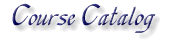MA - Mathematics back to catalog

MA101 College Algebra Three Credits
An Introductory College algebra course that provides an understanding of algetbraic processes and practical applications.
MA102 Applications of Fundamental Mathematics - Three Credits
An introductory study of mathematics at the college level, providing students with an understanding and application of math to everyday life
MA103 Introduction to Vedic Math - Three Credits
An introduction for non-science majors to ancient Vedic mathematicians, such as Aryabhata and Bhaskaracarya, and investigation into the originality of the Vedic system. Topics include: the Vedic number system, algebra, and trigonometry; Silpa-shastra geometry, including the Pythagorean theorem, used for ancient architecture; advanced calculus in Medieval India

back to catalog